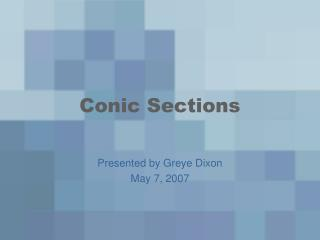DownloadDownload PresentationConic Sections

# Conic Sections

Download Presentation## Conic Sections

- - - - - - - - - - - - - - - - - - - - - - - - - - - E N D - - - - - - - - - - - - - - - - - - - - - - - - - - -
##### Presentation Transcript

1. Conic Sections Presented by Greye Dixon May 7, 2007

2. What are conic sections? • Conic sections are lines that define where a flat plane intersects with a double cone, which consists of two cones that meet at one another’s tip.

3. How can a conic section be drawn? • A conic section is shown as a graph. It can be shown as a circle, hyperbola, parabola, or ellipse. • Each section has its own formula for graphing. • As with all graphing, you use an in-and-out table.

4. Circles • Circles are the easiest to figure out and graph out of the four conic sections. • The formula for the radius of a circle is x2 + y2 = r2, with (0,0) as the center point of the circle. • The standard form for a circle is r2= ( x - h )2 + ( y - k )2

5. Parabolas • The formulas for a parabola with its origin at (0,0) are x2 = 4py for a vertical parabola, and y2 = 4px for a horizontal one. • It has a directrix, which is a line that is perpendicular to the parabola’s axis on the opposite side of the line that the parabola lies on. • It also has a fixed point, called a focus, which is the same distance from the vertex of the parabola as the directrix is. • In a vertical parabola, the focus is at (0,p). In a horizontal parabola, the focus is at (p,0).

6. Here are graphs of the two types of parabolas:

7. Hyperbolas • Hyperbolas are possibly the most difficult part of conic sections, due to both length and complexity. • The formulas for hyperbolas are • When x comes first, it is a horizontal transverse axis. • When y comes first, it is a vertical transverse axis. • The vertex is a units from the center, and the foci are c units from the center. The formula for c is c2 = a2 + b2. • The formula for asymptotes, the lines that decide the boundaries of the hyperbolas, is x = ± (b/a)y.

8. Here are the graphs of the two types of hyperbolas:

9. Ellipses • The last of the conic sections are ellipses. Ellipses are ovals, so the radii in different points are not completely identical. • The standard form of a ellipse is , depending on whether it’s horizontal or vertical. When the a comes first, it’s horizontal. When the b comes first, it’s vertical. • The center can be determined by the formula b2= a2 - c2. • The foci are either (±c,0), or (0,±c), depending on whether it’s vertical or horizontal.

10. Here arethe graphs of the two types of ellipses:

11. How are conic sections used in the real world? • Believe it or not, conic sections really can be used in real-world situations. The circle formula can be used to figure out how long it takes for the blast from a supernova to reach out to certain distances in space. • The ellipse formula can be used to find out the length and width of a running track. • The hyperbola formula can be used to figure out the angles of light coming from a lighthouse. • Parabolas can be used to measure things like suspension bridges.

12. Peer Assessment A directrix is a part of which type of conic section? a) ellipses b) parabolas c) hyperbolas d) circles If the radii are symmetrical, it is a: a) ellipse b) circle c) either d) none of the above

13. Peer assessment continued The only conic section with a minus sign in the formula is a: a) hyperbola b) circle c) parabola d) ellipse In a parabola the focus is: a) further from the vertex than the directrix b) closer to the vertex than the directrix c) equidistant from the vertex as the directrix d) there is no directrix in a parabola

14. Sources All pictures and information came from the following: • Thinkquest.org • Documents.wolfram.com • www.answers.com